# JP5385555B2 - Biopsy system, biopsy device, and biopsy method - Google Patents

## Info

Publication number
JP5385555B2
JP5385555B2 JP2008161048A JP2008161048A JP5385555B2 JP 5385555 B2 JP5385555 B2 JP 5385555B2 JP 2008161048 A JP2008161048 A JP 2008161048A JP 2008161048 A JP2008161048 A JP 2008161048A JP 5385555 B2 JP5385555 B2 JP 5385555B2
Authority
JP
Japan
Prior art keywords
fingers
related
acceleration
maximum value
opening
Prior art date
Legal status (The legal status is an assumption and is not a legal conclusion. Google has not performed a legal analysis and makes no representation as to the accuracy of the status listed.)
Active
Application number
JP2008161048A
Other languages
Japanese (ja)
Other versions
JP2009136667A (en
Inventor

Original Assignee

Priority date (The priority date is an assumption and is not a legal conclusion. Google has not performed a legal analysis and makes no representation as to the accuracy of the date listed.)
Filing date
Publication date
Priority to JP2007295935 priority Critical
Priority to JP2007295935 priority
Application filed by 日立コンシューマエレクトロニクス株式会社 filed Critical 日立コンシューマエレクトロニクス株式会社
Priority to JP2008161048A priority patent/JP5385555B2/en
Publication of JP2009136667A publication Critical patent/JP2009136667A/en
Application granted granted Critical
Publication of JP5385555B2 publication Critical patent/JP5385555B2/en
Application status is Active legal-status Critical
Anticipated expiration legal-status Critical

## Images

•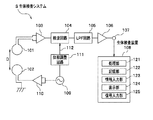•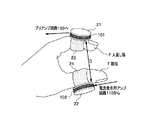•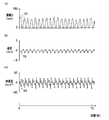••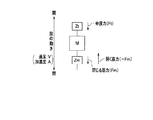•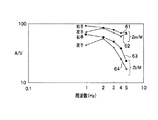•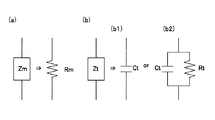•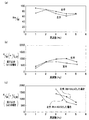•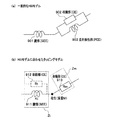•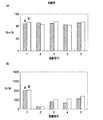•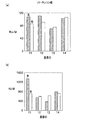•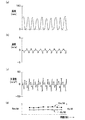•••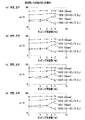•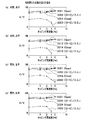••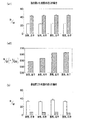## Classifications

• AHUMAN NECESSITIES
• A61MEDICAL OR VETERINARY SCIENCE; HYGIENE
• A61BDIAGNOSIS; SURGERY; IDENTIFICATION
• A61B5/00Detecting, measuring or recording for diagnostic purposes; Identification of persons
• A61B5/103Detecting, measuring or recording devices for testing the shape, pattern, colour, size or movement of the body or parts thereof, for diagnostic purposes
• A61B5/11Measuring movement of the entire body or parts thereof, e.g. head or hand tremor, mobility of a limb
• A61B5/1107Measuring contraction of parts of the body, e.g. organ, muscle
• AHUMAN NECESSITIES
• A61MEDICAL OR VETERINARY SCIENCE; HYGIENE
• A61BDIAGNOSIS; SURGERY; IDENTIFICATION
• A61B5/00Detecting, measuring or recording for diagnostic purposes; Identification of persons
• A61B5/22Ergometry; Measuring muscular strength or the force of a muscular blow
• A61B5/224Measuring muscular strength
• A61B5/225Measuring muscular strength of the fingers, e.g. by monitoring hand-grip force
• AHUMAN NECESSITIES
• A61MEDICAL OR VETERINARY SCIENCE; HYGIENE
• A61BDIAGNOSIS; SURGERY; IDENTIFICATION
• A61B5/00Detecting, measuring or recording for diagnostic purposes; Identification of persons
• A61B5/68Arrangements of detecting, measuring or recording means, e.g. sensors, in relation to patient
• A61B5/6801Arrangements of detecting, measuring or recording means, e.g. sensors, in relation to patient specially adapted to be attached to or worn on the body surface
• A61B5/6813Specially adapted to be attached to a specific body part
• A61B5/6825Hand
• A61B5/6826Finger
• AHUMAN NECESSITIES
• A61MEDICAL OR VETERINARY SCIENCE; HYGIENE
• A61BDIAGNOSIS; SURGERY; IDENTIFICATION
• A61B5/00Detecting, measuring or recording for diagnostic purposes; Identification of persons
• A61B5/68Arrangements of detecting, measuring or recording means, e.g. sensors, in relation to patient
• A61B5/6801Arrangements of detecting, measuring or recording means, e.g. sensors, in relation to patient specially adapted to be attached to or worn on the body surface
• A61B5/683Means for maintaining contact with the body
• A61B5/6838Clamps or clips
• AHUMAN NECESSITIES
• A61MEDICAL OR VETERINARY SCIENCE; HYGIENE
• A61BDIAGNOSIS; SURGERY; IDENTIFICATION
• A61B2562/00Details of sensors; Constructional details of sensor housings or probes; Accessories for sensors
• A61B2562/02Details of sensors specially adapted for in-vivo measurements
• AHUMAN NECESSITIES
• A61MEDICAL OR VETERINARY SCIENCE; HYGIENE
• A61BDIAGNOSIS; SURGERY; IDENTIFICATION
• A61B2562/00Details of sensors; Constructional details of sensor housings or probes; Accessories for sensors
• A61B2562/02Details of sensors specially adapted for in-vivo measurements
• A61B2562/0219Inertial sensors, e.g. accelerometers, gyroscopes, tilt switches
• AHUMAN NECESSITIES
• A61MEDICAL OR VETERINARY SCIENCE; HYGIENE
• A61BDIAGNOSIS; SURGERY; IDENTIFICATION
• A61B2562/00Details of sensors; Constructional details of sensor housings or probes; Accessories for sensors
• A61B2562/02Details of sensors specially adapted for in-vivo measurements
• A61B2562/0261Strain gauges
• AHUMAN NECESSITIES
• A61MEDICAL OR VETERINARY SCIENCE; HYGIENE
• A61BDIAGNOSIS; SURGERY; IDENTIFICATION
• A61B5/00Detecting, measuring or recording for diagnostic purposes; Identification of persons
• A61B5/40Detecting, measuring or recording for evaluating the nervous system
• A61B5/4076Diagnosing or monitoring particular conditions of the nervous system
• A61B5/4082Diagnosing or monitoring movement diseases, e.g. Parkinson, Huntington or Tourette
• AHUMAN NECESSITIES
• A61MEDICAL OR VETERINARY SCIENCE; HYGIENE
• A61BDIAGNOSIS; SURGERY; IDENTIFICATION
• A61B5/00Detecting, measuring or recording for diagnostic purposes; Identification of persons
• A61B5/45For evaluating or diagnosing the musculoskeletal system or teeth
• A61B5/4519Muscles
• AHUMAN NECESSITIES
• A61MEDICAL OR VETERINARY SCIENCE; HYGIENE
• A61BDIAGNOSIS; SURGERY; IDENTIFICATION
• A61B5/00Detecting, measuring or recording for diagnostic purposes; Identification of persons
• A61B5/45For evaluating or diagnosing the musculoskeletal system or teeth
• A61B5/4523Tendons

## Description

The present invention relates to a testing technique for cranial nerve diseases and the like.

In recent years, the number of patients with cranial nerve diseases such as Parkinson's disease and stroke has increased rapidly in Japan. For example, Parkinson's disease has four main symptoms: tremor, tremor (one of the conditions in which muscle tension is increased), postural reflex disorder (poor posture repositioning), and peristalsis (motion dullness). It is a cranial nerve disease. The rapid increase in patients with these cranial nerve diseases not only increases medical costs, but also causes great social losses due to their inability to work. Therefore, in order to solve such a social problem caused by a cranial nerve disease, it is necessary to determine whether or not the subject has a cranial nerve disease and, if it is a cranial nerve disease, the degree thereof with a certain degree of accuracy.

However, it is difficult to determine whether or not a subject has a cranial nerve disease and the degree thereof by a blood test or MRI (Magnetic Resonance Imaging) image findings. This is because the difference from the case of a healthy person is not always clear. Accordingly, it is often the case that whether or not a subject has a cranial nerve disease is subjectively determined by the experience and ability of a doctor who is an examiner. Against this background, for example, in order to evaluate motor function deterioration and rhythm abnormalities due to Parkinson's disease, a finger movement test for monitoring finger movement of hands has been performed.

As a method for monitoring the finger movement, for example, a method using an electric switch, a metal loop, a keyboard, a three-dimensional camera, or the like has been devised. However, these methods cannot be said to be simple methods and are not sufficiently widespread.
In view of this, the present applicant has proposed a biopsy device capable of detecting the movement of a part of a living body using a coil that generates magnetism (see Patent Document 1). According to the technique of Patent Document 1, it is possible to determine with high accuracy whether a patient is a cranial nerve disease patient or a healthy person by analyzing a waveform obtained from the movement of finger movement.
Japanese Patent No. 3841075

However, since the technique of Patent Document 1 does not dynamically analyze the finger movement of the subject, it is required to obtain an appropriate evaluation index regarding the hardness of the muscle used for the finger movement of the subject. There was a problem that I could not say.
Therefore, the present invention has been made in view of the above problems, and it is intended to dynamically analyze a subject's finger movement and obtain an appropriate evaluation index related to muscle hardness and the like used for the subject's finger movement. Let it be an issue.

According to the present invention, the processing unit of the biopsy device acquires or calculates the speed and acceleration of the two fingers based on the motion information of the two fingers obtained from the tapping detection device, and calculates the ratio of the speed and acceleration. The calculated ratio is applied to a predetermined calculation formula based on the muscle mechanics model, and the mechanical impedance related to the extension force of the two fingers and the mechanical impedance related to the opening / closing force of the two fingers are calculated as evaluation indexes. Other means will be described later.

ADVANTAGE OF THE INVENTION According to this invention, a test subject's finger motion can be analyzed dynamically and the suitable evaluation parameter | index regarding the hardness etc. of the muscle used for a test subject's finger motion can be calculated | required.

Hereinafter, the best mode for carrying out the present invention (hereinafter referred to as “embodiment”) will be described in detail with reference to the drawings (refer to drawings other than the referenced drawings as appropriate).

[First embodiment]
Hereinafter, first, a first embodiment of the present invention will be described.
<< Configuration >>
FIG. 1 is an overall configuration diagram of the biopsy system according to the first embodiment. Each configuration will be described in detail along the operational flow of the first embodiment.

First, an AC voltage having a specific frequency (for example, 20 kHz) is generated by the AC generation circuit 109. The AC voltage having a specific frequency generated by the AC generation circuit 109 is converted into an AC current having a specific frequency by the current generation amplifier circuit 110. Then, an alternating current generated by conversion by the current generation amplifier circuit 110 flows through the transmission coil 102. The magnetic field generated by the current flowing through the transmitting coil 102 generates an induced electromotive force in the nearby receiving coil 101. For example, the transmitting coil 102 is attached to the thumb of one hand of the subject, and the receiving coil 101 is attached to the index finger of the same hand of the subject. Details of this state will be described later with reference to FIG.

The induced electromotive force generated in the receiving coil 101 (the frequency is the same as the frequency of the AC voltage generated by the AC generation circuit 109) is amplified by the preamplifier circuit 103, and the amplified signal is input to the detection circuit 104. In the detection circuit 104, detection is performed at a specific frequency or a double frequency generated by the AC generation circuit 109. Therefore, the phase adjustment circuit 111 adjusts the phase of the output from the AC generation circuit 109 and then inputs the reference signal 112 to the reference signal input terminal of the detection circuit 104.

In addition, the phase adjustment circuit 111 is not necessarily required when detecting at a frequency twice the specific frequency. As a simple circuit configuration for detecting at a double frequency, for example, the specific frequency of the AC generation circuit 109 is set to a double frequency, converted to a half frequency by a division cycle, and then input to the current generation amplifier circuit 110. A signal having a frequency twice the specific frequency may be input to the reference signal input terminal of the detection circuit 104 as the reference signal 112.

An output signal from the detection circuit 104 passes through an LPF (Low-Pass Filter) circuit 105, is amplified by an amplifier circuit 106 to obtain a desired voltage, and is introduced into the biological examination apparatus 108 as an output signal 107. . The output signal 107 has a voltage corresponding to the distance D between the transmitting coil 102 and the receiving coil 101 mounted on the thumb and index finger, respectively. Note that, in the configuration described above, components other than the output signal 107 and the reference signal 112 correspond to a part of the “tapping detection device” in the claims.

The biopsy device 108 is a computer device that records and analyzes the output signal 107, and includes a processing unit 121, a storage unit 122, an information input unit 123, a display unit 124, and a signal input unit 125.
The processing unit 121 dynamically analyzes the subject's finger movement based on the output signal 107, and displays the analysis result on the display unit 124. The processing unit 121 is realized by, for example, a CPU (Central Processing Unit). The

The storage unit 122 is a storage device or a temporary storage device for storing various programs, data, analysis results, and the like, and includes, for example, a ROM (Read Only Memory), a RAM (Random Access Memory), and a hard disk. The processing unit 121 can execute various operations by expanding the program, data, and the like stored in the storage unit 122 in the storage unit 122, and can store data, analysis results, and the like in the storage unit 122.

The information input unit 123 is used by the operator of the biopsy apparatus 108 to input information about the subject, and is configured from, for example, a keyboard and a mouse. Further, the information input unit 123 may have a GUI (Graphical User Interface) function.
The display unit 124 displays data generated by the processing unit 121, analysis results, and the like, and includes, for example, an LCD (Liquid Crystal Display), a CRT (Cathode Ray Tube) display, or the like.
The signal input unit 125 is an interface that receives an input of the output signal 107 from the amplifier circuit 106.

FIG. 2 is an explanatory diagram showing a state where the transmitting coil 102 and the receiving coil 101 are attached to one hand (here, the left hand) of the subject in the first embodiment.
As shown in FIG. 2, the transmitting coil 102 connected to the current generating amplifier circuit 110 is wound around the coil mounting member 22, and the band portion 24 bonded to the coil mounting member 22 is attached to the thumb T. Thus, the coil mounting member 22 and the transmitting coil 102 are fixed to the thumb T.

Similarly, the receiving coil 101 connected to the preamplifier circuit 103 is wound around the coil mounting member 21, and the band portion 23 bonded to the coil mounting member 21 is attached to the index finger F. The receiving coil 101 is fixed to the index finger F.
The band portions 23 and 24 are preferably made of rubber, sponge, or the like and can absorb individual differences in finger size (thickness).

With such a configuration, the relationship between the distance D between the thumb T and the index finger F and the voltage of the output signal 107 is stored in the storage unit 122 of the biopsy device 108 in advance, whereby the voltage of the output signal 107 is stored. The distance D can be specified from The finger that fixes the transmitting coil 102 and the receiving coil 101 is not limited to the thumb T or the index finger F, and may be any finger.

FIG. 3 shows an example of information obtained by tapping (opening / closing operation of two fingers (thumb T and any one of the remaining fingers)), and (a) shows time and two fingers (thumb T and index finger F). FIG. 4B is a relationship diagram of time and the relative speed (velocity) of two fingers, and FIG. 4C is a relationship diagram of time and the relative acceleration (acceleration) of two fingers. Hereinafter, the “maximum value” and the “minimum value” are collectively expressed as “maximum value”. For this reason, the term “local minimum” is not used in this specification. By the way, “local maximum of function (that is, the maximum or minimum value in the vicinity of a certain point)” is called “maximum value, minimum value” respectively, and these are collectively called “extreme value”. . In the present embodiment, the maximum value in a predetermined section of data obtained in time series is the maximum value (the minimum value is the minimum value).

As shown in FIG. 3A, a waveform 31 (distance waveform) represents a time change of the distance D (mm) between two fingers, and a portion indicated by a white circle (◯) in the drawing (a valley portion of the waveform). Represents a moment when the distance D is “0”, that is, when two fingers are attached. The waveform 31 is generated when the processing unit 121 of the biological examination apparatus 108 converts the voltage value of the output signal 107 into a distance.

As shown in FIG. 3 (b), the waveform 32 (velocity waveform) represents the time change of the relative speed (m / s) of two fingers, and the two fingers open in the portion indicated by a cross (+) in the figure. It represents the positive peak (maximum value) of the hour (opening) speed, and the white circle (○) in the figure represents the negative peak (maximum value) of the speed when the two fingers are closed (closing). Represents. That is, the speed is the same for the acceleration with the direction of the opening being the positive direction. The waveform 32 is generated when the processing unit 121 of the biopsy apparatus 108 performs time differentiation on the waveform 31 of the distance D between two fingers. By the way, the speed becomes 0m / s when the two fingers are stuck. Similarly, the speed becomes 0 m / s even when the two fingers are fully opened.

As shown in FIG. 3 (c), the waveform 33 (acceleration waveform) represents the time change of the relative acceleration (m / s2) of the two fingers, and the portion indicated by a cross (+) in the figure is the acceleration of the opening. The positive peak time is shown, and the portion indicated by a white circle (◯) in the figure shows the negative peak time of the closing acceleration. The waveform 33 is generated when the processing unit 121 of the biological examination apparatus 108 differentiates the waveform 32 with respect to time.

Hereinafter, these distance waveform, velocity waveform, and acceleration waveform are collectively referred to as “motion waveform”. Even if a strain gauge, an accelerometer, or the like is applied instead of the transmission coil 102 and the reception coil 101, if at least one motion waveform is measured, the other integral is calculated by performing a calculus operation. A motion waveform can be obtained. Further, since details of generation of the motion waveform are described in detail in Patent Document 1, further detailed description is omitted here.

<< Kinematic analysis model >>
Next, the kinematic analysis model of the first embodiment will be described. In the following, the mechanical impedance is a quantity indicating the difficulty of movement of an object (here, a finger), the mass of the object, mechanical resistance (friction etc.) when the object is operated, and a spring constant (here, a tendon is This is an amount determined depending on the spring). The name is given because it corresponds to the impedance in an electric circuit.

Consider the following two simple equations (Equation (1) and Equation (2)) using the formula of finger kinematics considering mechanical impedance and the law of inertia.

Here, F (N = kg · m / s2) is the force acting on the finger, Z (kg / s) is the finger mechanical impedance, M (kg) is the finger mass, and A (m / s2) is the finger acceleration. , V (m / s) is the finger speed.

By combining these expressions (1) and (2), the following expression (3) can be derived.

From the expression (3), it can be seen that the mechanical impedance (Z / M) normalized by the mass of the finger can be evaluated by using the value of A / V. It is considered that this standardization can absorb individual differences such as finger size. In the first embodiment, model analysis is performed using the value of A / V. That is, since the value of Z / M can be obtained indirectly by obtaining the value of A / V, the mechanical impedance (Z) and the mass (M) of the finger are not directly measured or calculated. A value of Z / M can be obtained.

In order to evaluate the A / V value, the tapping opening speed and acceleration maximum values (hereinafter referred to as maximum speed and maximum acceleration), and the closing maximum speed and acceleration are determined for all tapping cycles. Thus, extraction is performed as shown in FIGS. Then, the average value of each extracted maximum speed and each maximum acceleration is calculated. Here, the opening average maximum velocity is Vo, the opening average maximum acceleration is Ao, the closing average maximum velocity is Vc, and the closing average maximum acceleration is Ac.

(Measuring method)
Here, in order to assist in understanding the analysis model, an example of a measurement method and an analysis result thereof will be described.
When measuring the finger movement, the subject wears the transmitting coil 102 on the thumb T and the receiving coil 101 on the index finger F as shown in FIG. 2 and performs tapping in that state. Measure the distance between. Instead of the band portions 23 and 24, a medical double-sided tape or the like may be used so that the transmitting coil 102 is attached to the nail of the thumb T and the receiving coil 101 is attached to the nail of the index finger F. Good. Further, the arrangement of the transmitting coil 102 and the receiving coil 101 may be either the thumb T or the index finger F, and the attachment position is not limited to the position of the nail.

Then, a 20 kHz magnetic field is generated from the transmitting coil 102 and the electromotive force induced in the receiving coil 101 is detected. Next, the distance between the fingers is measured by converting the output obtained by lock-in detection of the detected electromotive force at 20 kHz (detecting only the component of 20 kHz (near)). Details of these operations are as described above with reference to FIG.

The subject performs tapping in a relaxed sitting position. There are two types of inspections: a periodic inspection that uses a metronome to perform tapping according to the timing of 1, 2, 3, 4, 5 Hz, etc., and an aperiodic inspection that performs tapping as quickly as possible (frequency is about 2 to 5 Hz) There is).

4A and 4B are diagrams showing analysis results (average of three persons) of finger movements measured by the periodic inspection, where FIG. 4A shows the analysis result of the right hand and FIG. 4B shows the analysis result of the left hand. As shown in FIGS. 4 (a) and 4 (b), the A / V values (reference numerals 401 and 411) of the opening (Open) are relatively large at all frequencies in both the right hand (all three dominant hands) and the left hand. As the frequency increases, the closing A / V values (reference numerals 404 and 414) increase, while the opening A / V values (reference numerals 401 and 411) tend to decrease.

Also, from these tendencies, the value [(O + C) / 2] (reference numerals 402, 412) obtained by adding the closing and opening A / V values and dividing by 2 is a constant value without depending on the frequency. I understand that. On the contrary, the value [(OC) / 2] (reference numerals 403 and 413) obtained by subtracting the closing A / V value from the opening and dividing by 2 is the A / V value as the frequency increases. It can be seen that is decreasing. These tendencies are common to both right and left hands.

<Tapping muscle mechanics model (analysis model)>
A tapping muscle mechanics model will be described with reference to the relationship between the value of A / V in FIG. 4 and the frequency.
First, it is assumed that the fingertip has a mass M and opens and closes at a speed V and an acceleration A. The operation of the mass M is considered to be composed of a balance between the mechanical impedance Zt that affects the extension force (Ft) and the mechanical impedance Zm that affects the opening / closing force (Fm). Here, it is considered that the force Ft always pushes the mass M of the fingertip in the direction in which the fingers are closed (the fingers approach each other). In such a model, it is considered that Zt is a mechanical impedance determined mainly based on an extensor tendon (one of finger tendons) and Zm mainly based on a worm-like muscle (one of finger muscles). It is done.

These are schematically shown in FIG. FIG. 5 is a schematic diagram showing a tapping muscle dynamic model. Since it is considered that the force Ft always pushes the mass M of the fingertip in the direction of closing the finger, the muscular force for opening the finger (−Fm) requires a larger force than the muscular force for closing the finger (Fm).

When the finger moves in the opening direction, the following equation (4a) is established, and when it is deformed using equation (1), equation (4b) is obtained, and when further equation transformation is performed, equation (4) is obtained.

Further, when the finger moves in the closing direction, the following formula (5a) is established, and if the formula is deformed using formula (1), formula (5b) is obtained, and if the formula is further transformed, formula (5) is obtained.

When equations (4) and (5) are calculated simultaneously, the following equations (6) (predetermined equation) and (7) (predetermined equation) can be obtained.

Using equations (6) and (7), the values of Zm / M and Zt / M can be obtained (estimated) from the values of Ao, Ac, Vo and Vc.

When the values of Zm / M and Zt / M are calculated using equations (6) and (7), the results shown in FIG. 6 are obtained. FIG. 6 is a diagram showing the values of Zm / M and Zt / M for each frequency.
As shown in FIG. 6, it can be seen that Zm / M (reference numerals 61 and 62) has a small frequency dependence and is close to a constant value. On the other hand, it can be seen that Zt / M (reference numerals 63 and 64) rapidly decreases as the frequency increases.

From the calculation results shown in FIG. 6, it is considered that Zm is mainly dominated by mechanical resistance (Rm), and Zt is mainly compliance (Ct (elasticity and deflection strength when external force is applied): 1 / Ct is It can be considered that it reflects the frequency characteristics due to the spring constant Kt (of the tendon). Based on this idea, an equivalent circuit diagram of Zm and Zt is considered as shown in FIG. In the following, when each parameter is normalized by mass, each parameter may be divided by M.

7A is an equivalent circuit diagram relating to Zm, and FIG. 7B is an equivalent circuit diagram relating to Zt. Regarding Zt in (b), since it has a characteristic that decreases with frequency and a characteristic that is slightly flat between 1 Hz and 2 Hz, parallel connection of Ct and Rt ((b2) in FIG. 7B) is also considered. Therefore, a parallel connection model will also be studied.
As shown in FIG. 7A, Zm is expressed by the following equation (8).

Further, when Zt is approximated only by Ct ((b1) in FIG. 7B), Equation (9) is obtained, and the magnitude of Zt is expressed by Equation (10). Here, j is an imaginary unit, and ω is an angular frequency.

When Zt is approximated by parallel connection of Ct and Rt ((b2) in FIG. 7B), in order to simplify the calculation, if calculation is performed with admittance (Yt = 1 / Zt) that is the inverse of Zt. , Yt and their sizes are as shown in equations (11) and (12).

FIG. 8 shows Rm / M and Kt / M (= 1 / CtM) obtained by calculation and normalization using the equations (8), (10), and (12). As shown in FIG. 8 (a), Rm / M has a tendency to decrease slightly with the frequency in both the right hand and the left hand, but is almost constant, and it can be seen that the value is between “70” and “80”. .

Regarding Kt / M, the calculation using only Ct ((b1) in FIG. 7B) is a small value of “400” or less in the vicinity of 1 Hz close to a flat characteristic, but from “500” after 2 Hz. It can be seen that the value is “1000” and a relatively stable value is obtained (FIG. 8B).

On the other hand, in the parallel connection calculation ((b2) in FIG. 7B), 1 / CtM is calculated on the assumption that the Zt / M value of 1 Hz, which is a flat characteristic, is the Rt / M value as it is. ing. It can be seen that the calculated value of 1 / CtM greatly decreases as the frequency increases (FIG. 8C).

Here, the calculation is simply performed as described above. However, as a method of calculating the values of Rt and Ct, it is also possible to calculate using two measured points (for example, values of 1 Hz and 3 Hz). However, when calculation was performed in conformity with actual data, a solution could not be obtained due to incompatibility between the model and the actual measurement value. From the above, it is considered most appropriate to consider Zt by approximation of Ct only at 2 Hz or higher.

<Summary>
As a tapping muscular mechanics model, it is assumed that the mechanical impedance Zm related to the extension force of the two fingers is mainly the mechanical resistance Rm of the muscles that control the opening and closing of the fingers such as the worm-like muscles. Assuming that the compliance Cm (1 / Kt) plays the role of a spring represented by an extensor tendon is suitable for a tapping muscle mechanics model.

From the results estimated in this way, it is found that Rm / M is between about “70” and “80” and the value of Kt / M is between about “500” and “1000”. The possibility that it is. Therefore, by creating a database of healthy individuals regarding the values of Rm / M and Kt / M, the values of Rm / M (= Zm / M) and Kt / M (= M / Zt) It can be considered as an evaluation index.

The estimated Rm / M and Kt / M are Hill models (Delp S, Loan P, Hoy M, Zajac FE, Fisher S, and Rosen J. An interactive graphics-based model of the lower extremity to study orthopaedicsurgical procedures. IEEE Trans. on Biomedical Engineering, 37 (8), August 1990, 757-767.)) is very close to the muscle model (Fig. 9 (a)). As shown in FIG. 9A, the contraction portion 902 and the parallel elastic portion 903 (the coiled shape means springiness) are connected in parallel, and the tendon portion 901 is connected in series. It is connected.

As an example, FIG. 9B shows a model obtained by replacing the current term with general terms used in the Hill model. It is considered that Rm corresponds to a contraction element (CE) 913, Rt corresponds to a contraction part 912, and Kt corresponds to a tendon part (series elastic element: SEE) 911. That is, the tendon portion 911 and the contraction portion 912 correspond to Zt (see (b2) in FIG. 7B), and the contraction portion 913 corresponds to Zm (see FIG. 7A).

As described above, the tapping muscle mechanics model in the first embodiment is similar to the Hill model and can be said to have high relevance. However, the analysis method using the tapping muscle mechanics model in the first embodiment uses the law of inertia or the like to determine the hardness of the muscle used for finger movement from the speed and acceleration during opening and closing. Therefore, it has novelty and inventive step even when compared with the Hill model.

<< Experimental data >>
Next, with reference to FIG. 10 to FIG. 12, data of an experiment by an aperiodic examination regarding the tapping muscle dynamic model of the first embodiment will be described. FIG. 10 is data relating to an experiment conducted on five healthy elderly persons (hereinafter, also simply referred to as “elderly persons”) who have no history of brain disease or the like as subjects. In FIG. 10A, the horizontal axis represents the elderly ID, and the vertical axis represents the value of Rm / M (for each elderly person, the left bar graph is the left hand and the right bar graph is the right hand). Similarly, in FIG. 10B, the horizontal axis indicates the elderly person ID, and the vertical axis indicates the value of Kt / M.

Similar to the case of FIG. 10, FIG. 11 is data relating to an experiment conducted with four Parkinson's disease patients as subjects. In FIG. 11A, the horizontal axis represents the patient ID, and the vertical axis represents the value of Rm / M. Similarly, in FIG. 11B, the horizontal axis represents the patient ID, and the vertical axis represents the value of Kt / M.

As can be seen by comparing FIG. 10 and FIG. 11, both the values of Rm / M and Kt / M tend to be higher in patients with Parkinson's disease. Therefore, for the values of Rm / M and Kt / M, for example, data of many healthy elderly people are collected and averaged, and how much higher than the average value is used for finger movement. It can be used as an evaluation index for muscle hardness or the like. Note that normalization by dividing by the finger mass M is not essential.

Specifically, a value converted into a value normalized by a calculation formula “standard normal distribution value = (measured value−average value) / standard deviation” can be calculated as an evaluation index. Here, “average value” indicates an average value of values measured in healthy elderly persons, and “standard deviation” indicates a standard deviation of values measured in healthy elderly persons (plural persons). Moreover, you may produce the said evaluation parameter | index regarding the other parameter obtained from each motion waveform besides the value of Rm / M and the value of Kt / M.

By using this standard normal distribution value (normalized value) as an evaluation index, it is possible to quantitatively understand how much the measured data deviates from the standard value (average value). That is, if the value is the same as the average value, the value is “1”, if the value is small, the value is smaller than the standard value (for example, “0.8”), and if the value is large, the value is larger than the standard value (for example, “1.3”). ]), It can be easily understood by elderly people and the like. The values of these evaluation indexes are displayed on the display unit 124 of the biopsy device 108, and the evaluation criteria can be presented to the examiner and the subject.

Alternatively, the Rm / M value and the Kt / M value may be calculated and displayed for each tapping. FIG. 12 is an explanatory diagram for the case where the value of Rm / M and the value of Kt / M are calculated for each tapping. 12 (a) to 12 (c) are the same as FIGS. 3 (a) to 3 (c), and a description thereof will be omitted. As shown in FIG. 12D, the value of Rm / M and the value of Kt / M can be calculated and displayed for each tapping. In this way, for example, the value of Rm / M and the value of Kt / M do not change significantly even after 20 seconds have passed in healthy subjects, but the value of Rm / M and Kt have not changed much in Parkinson's disease patients etc. after 20 seconds have passed. An evaluation index such as muscle hardness used for finger movement can be calculated and used based on a difference between a healthy person and a Parkinson's disease patient or the like such that the value of / M changes greatly.

Thus, according to the biopsy system S of the first embodiment, it is possible to dynamically analyze the finger movement of the subject and obtain an evaluation index such as the hardness of the muscle used for the finger movement. That is, for example, Parkinson's disease patients are often in a state where the muscles are in force at all times, and the muscles tend to stiffen easily, as in the first embodiment. By performing an analysis based on the muscle mechanics model, an appropriate evaluation index such as muscle hardness can be obtained.

This is the end of the description of the first embodiment, but aspects of the present invention are not limited to these.
For example, the present invention is not only applied to the examination of Parkinson's disease but also can be effectively used for examination of other cranial nerve diseases such as cerebral infarction and degenerative diseases such as rheumatism.
In addition, specific configurations of hardware, programs, and the like can be appropriately changed without departing from the gist of the present invention.

[Second Embodiment]
Next, a second embodiment of the present invention will be described. The difference from the first embodiment is that the finger movement is close to the state where the two fingers are fully opened (hereinafter referred to as “the vicinity of the open state”) and the two fingers are closed (the two fingers are closed). The finger movement in the vicinity of the touched state (hereinafter referred to as “near the contact state”) is handled separately, and more detailed modeling and calculation are performed for the movement of the two fingers.

<< Configuration >>
Since the configuration of the biopsy system of the second embodiment is the same as the configuration of the biopsy system of the first embodiment (FIGS. 1 and 2), description thereof is omitted.

FIG. 13 is an example of information obtained by tapping (opening / closing operation of two fingers (thumb T and one of the remaining one fingers)). FIGS. 13A to 13C are diagrams in which the same diagram as FIGS. 3A to 3C is enlarged in the time axis direction, and matters common to FIGS. 3A to 3C are described. Description is omitted.

As shown in FIGS. 13A to 13C, each tapping can be divided into an opening section 134 and a closing section 135. The boundary between the opening section 134 and the closing section 135 is a point P1 (the point of the maximum value of the distance D (mm) between two fingers) shown in FIG. In the time zone of the opening section 134, the subject performs a two-finger opening operation, and in the time zone of the closing section 135, the subject performs a two-finger closing operation.

In FIG. 13 (b), point OP1 indicates a positive peak (maximum value) when the two fingers open (opening), and point CP1 indicates a negative speed when the two fingers close (closing). The peak (maximum value) is shown.

In FIG. 13 (c), the point OP2 indicates the time of the positive peak (maximum value) of the acceleration of the opening (open motion section), and the point OP3 indicates the time of the negative peak (maximum value) of the acceleration of the opening. Yes.
In FIG. 13 (c), point CP2 indicates the time of a positive peak (maximum value) of closing (closed motion section) acceleration, and point CP3 indicates the time of a negative peak (maximum value) of closing acceleration. Yes.

Here, in the time zone of the zone including the opening zone 134 and the closing zone 135, for example, the time zone from point OP1 to point CP1 shown in FIG. It is a time slot | zone which is included, and the other time slot | zone is a time slot | zone including the motion in the contact state vicinity of two fingers. In the second embodiment, the values of Vo, Ao, Vc and Ac are calculated and used for each time zone. Here, the values at points OP1, OP3, CP1, and CP3 are used as Vo, Ao, Vc, and Ac in the motion near the open state of two fingers (see Table 1). Further, the values at points OP1, OP2, CP1, and CP2 are used as Vo, Ao, Vc, and Ac in the motion in the vicinity of the two-finger contact state (see Table 1). Incidentally, in the second embodiment, Vo is the average maximum speed of opening, Ao is the average maximum acceleration of opening, Vc is the average maximum speed of closing, and Ac is the average maximum acceleration of closing.

<< Kinematic analysis model >>
Next, the kinematic analysis model of the second embodiment will be described. Note that description of matters similar to those in the first embodiment is omitted as appropriate. Formula (1), Formula (2), Formula (3), and the premise thereof are the same as in the first embodiment.

As in the first embodiment, in order to evaluate the A / V value, the maximum speed and maximum acceleration at the opening of tapping, and the maximum speed and maximum acceleration at closing are shown in FIG. Extract as shown in b) and (c).

FIG. 14 is an explanatory diagram of a kinematic analysis model of the second embodiment. FIG. 14A is an explanatory diagram in the vicinity of the open state, FIG. 14A is a schematic diagram of a subject's hand, and FIG. 14A is a dynamic model diagram.

As shown in FIG. 14 (a1), when tapping, both the thumb T and the index finger F actually move, but here, for simplicity of explanation, the thumb T is fixed and only the index finger F moves. And Further, in order to easily model the actual human hand structure, it is assumed that the index finger F has a bone 140, a flexor muscle Q 1 , and an extensor muscle Q 2 .

The flexor muscle Q 1 contracts according to the intention of the subject, and plays the role of bringing the index finger F closer to the thumb T. The extensor muscle Q 2 plays a role of moving the index finger F away from the thumb T by contracting according to the intention of the subject.

F 1 represents the force produced by the flexor Q 1, the resultant force of the spring force by the tendon which is connected to the flexor Q 1 (hereinafter, referred to as "flexor Q 1 side force".) The. F 2 represents a shrinkage force produced by the extensor muscle Q 2, the resultant force of the spring force by the tendon which is connected to the extensor muscle Q 2 (hereinafter, referred to as "extensor Q 2 side of the power".) The. Further, F 1 and F 2 have values of “0” or more, and the opening direction of two fingers is a positive direction.

Here, in the vicinity of the open state, when the subject weakens, the two fingers approach naturally, and when the two fingers are opened, a repulsive force is generated by the tendon of the flexor Q 1 in addition to the contraction force of the extensor Q 2. It is assumed that when the two fingers are closed, an urging force (supporting force) is generated by the tendon of the extensor Q 2 in addition to the contraction force of the flexor Q 1 .

That is, as shown in FIG. 14 (a2), if you open the two fingers in the open state near the force F 2 extensor Q 2 side becomes the driving force, and Ko' the force -F 1 flexor Q 1 side, Assume that two fingers open. In this case, the equation (13a) is established as an equation of motion, and when the equation (13a) is transformed using the equation (1), the equation (13b) is obtained, and when this equation (13b) is further transformed, the equation (13) is obtained.

Further, as shown in FIG. 14 (a2), if in the open state near closing two fingers, force -F 1 flexor Q 1 side becomes the driving force, further force is applied -F 2 extensor Q 2 side, Assume that two fingers close. In this case, the equation (14a) is established as an equation of motion, and when the equation (14a) is transformed using the equation (1), the equation (14b) is obtained, and when this equation (14b) is further transformed, the equation (14) is obtained.

When the equations (13) and (14) are calculated simultaneously, the following equations (15) (calculation equations for the open motion near the open state) and (16) (calculation equations for the close motion near the open state) ) Can be obtained.

By using the equations (15) and (16), the values of Z 1 / M and Z 2 / M can be obtained (estimated) from the values of Ao, Ac, Vo and Vc in the vicinity of the open state. In this case, Z 1 / M represents the mechanical impedance related to the closing motion of the two fingers, and Z 2 / M represents the mechanical impedance related to the opening motion of the two fingers.

On the other hand, in the vicinity of the contact state, when the subject weakens, the two fingers naturally move away, and when the two fingers are opened, the urging force by the tendon of the flexor Q 1 is generated in addition to the contraction force of the extensor Q 2. It is assumed that when the two fingers are closed, a repulsive force is generated by the tendon of the extensor Q 2 in addition to the contraction force of the flexor Q 1 .

That is, as shown in FIG. 14 (b2), if in contact with the vicinity of opening the two fingers, the force F 2 extensor Q 2 side becomes the driving force, further force is applied F 1 flexor Q 1 side, two fingers Shall be opened. In this case, the equation (17a) is established as an equation of motion, and when the equation (17a) is transformed using the equation (1), the equation (17b) is obtained, and when this equation (17b) is further transformed, the equation (17) is obtained.

Further, as shown in FIG. 14 (b2), to close the two fingers in contact near the force -F 1 flexor Q 1 side becomes the driving force, and Ko' the force F 2 extensor Q 2 side, Assume that two fingers close. In this case, the equation (18a) is established as an equation of motion, and when the equation (18a) is transformed using the equation (1), the equation (18b) is obtained, and when this equation (18b) is further transformed, the equation (18) is obtained.

When the equations (17) and (18) are calculated simultaneously, the following equations (19) (calculation equations for the open motion near the contact state) and (20) (calculation equations for the close motion near the contact state) ) Can be obtained.

If Expressions (19) and (20) are used, the values of Z 1 / M and Z 2 / M can be obtained (estimated) from the values of Ao, Ac, Vo and Vc in the vicinity of the contact state.

FIG. 15 is a diagram showing analysis results (average of a plurality of persons) of finger movements measured by the periodic inspection for movements in the vicinity of the open state of two fingers (near the state where the two fingers are opened). ) Shows the analysis result of the female left hand, (b) shows the analysis result of the female right hand, (c) shows the analysis result of the male left hand, and (d) shows the analysis result of the male right hand. Note that both ends of the shape of “I” given to each value indicate the standard deviation of each value. As a result, it can be seen that most values are reliable data with a small standard deviation, that is, little variation.

As shown in FIG. 15A, each value of reference numeral 1001 is an A / V value of opening (Open), and each value of reference numeral 1002 is a value obtained by adding the closing and opening A / V values and dividing by two. [(C + O) / 2] (Z 2 ), each value of 1003 is the value obtained by subtracting the opening A / V value from the closing A / V value and dividing by 2 [(C−O) / 2] Each value of (Z 1 ) and reference numeral 1004 indicates an A / V value of closing.

As seen from FIG. 15 (a), the value of Z 2 are substantially constant value regardless of the frequency, the value of Z 1 tends to decrease with frequency from per frequency 2～3Hz. Therefore, when each mechanical impedance is replaced with an electric circuit, as shown in FIG. 17, Z 1 can be considered as a parallel circuit of a resistor R and a capacitor C (see (a1)), and Z 2 is considered as an electric resistance R. (See (a2)). FIG. 17 is an equivalent circuit diagram similar to FIG.

FIG. 15 (b), the in (c) and (d), reference numerals 1011 to 1014, code 1021 to 1024 and reference numeral 1031 to 1034 corresponds to the code 1011 to 1014 in FIG. 15 (a), the and Z 1 Z for two, as shown in FIG. 15 (a) (not the difference was observed due to gender differences and the right hand left hand) a similar trend can be seen with.

FIG. 16 is a diagram showing an analysis result (average of a plurality of persons) of finger movements measured by a periodic inspection for movements near the contact state of two fingers (near the state where the two fingers are closed); ) Shows the analysis result of the female left hand, (b) shows the analysis result of the female right hand, (c) shows the analysis result of the male left hand, and (d) shows the analysis result of the male right hand.

As shown in FIG. 16 (a), each value of reference numeral 2001 is an A / V value of opening (Open), and each value of reference numeral 2002 is a value obtained by adding the opening and closing A / V values and dividing by two. [(O + C) / 2] (Z 1 ), each value of reference numeral 2003 is a value obtained by subtracting the closing A / V value from the opening A / V value and dividing by 2 [(O−C) / 2]. Each value of (Z 2 ) and reference numeral 2004 indicates an A / V value of closing.

As can be seen from FIG. 16 (a), the value of Z 1 is substantially constant value regardless of the frequency, the value of Z 2 may have little value in the above 4Hz becomes a constant value until position 3 Hz. Therefore, when each mechanical impedance is replaced with an electric circuit, both Z 1 and Z 2 ( 1 to 3 Hz) can be considered as electric resistance R as shown in FIG. 17 (see (b1) and (b2)).

FIG. 16 (b), the in (c) and (d), reference numerals 2011 to 2014, code 2021 to 2024 and reference numeral 2031 to 2034 corresponds to the code 2011 to 1024 in FIG. 16 (a), the and Z 1 Z for two, (is not observed differences by gender differences and the right hand left hand) a similar trend can be seen as in FIG. 16 (a).

<< Experimental data >>
Next, the data of the experiment of the second embodiment will be described with reference to FIG. The test subjects are middle-aged and healthy men and women who have no history of brain disease. 18 (a1) and (a2) are data relating to the movement of the two fingers near the open state, and FIG. 18 (b) is data relating to the movement of the two fingers in the vicinity of the contact state (both data have a frequency of about 2 Hz). Of the case).

In FIG. 18 (a1), the horizontal axis represents the left women from left to right, right, left male right hand, and the vertical axis represents the value of R / M (white bars correspond to Z 1. Hatched bar graphs Z 2 Corresponding). In FIG. 18A2, the horizontal axis is the same as in FIG. 18A1, and the vertical axis indicates the value of K / M (same as in FIG. 10B).

In FIG. 18 (b), the horizontal axis is the same as that of FIG. 18 (a1), the vertical axis represents the value of R / M (white bars correspond to Z 1. Hatched bars correspond to Z 2) shows .
In FIGS. 18A1 and 18B, the upper end of the shape of “T” attached to each value indicates the standard deviation of each value. As a result, it can be seen that most values are reliable data with a small standard deviation, that is, little variation.

As shown in FIG. 18, according to the biopsy system S of the second embodiment, the finger movement of the subject is dynamically analyzed, and an appropriate evaluation index relating to the hardness of the muscle used for the finger movement of the subject. In addition, the characteristics of each value relating to the motion in the vicinity of the open state and the contact state of the two fingers can be evaluated separately. In other words, deriving parameters (each value) for more accurately grasping diseases that tend to cause abnormalities near the contact state (for example, Parkinson's disease) and diseases that tend to cause abnormalities near the open state (for example, stroke). Can do.

This is the end of the description of the second embodiment, but aspects of the present invention are not limited to these.
For example, as in the first embodiment, each mechanical impedance can be normalized by dividing by the mass of two fingers, or normalized by the respective average value and standard deviation, and used as an evaluation index.
In addition, specific configurations of hardware, programs, and the like can be appropriately changed without departing from the gist of the present invention.

It is a whole lineblock diagram of the living body inspection system of this embodiment. In this embodiment, it is explanatory drawing which shows a mode that the coil for transmission and the coil for reception were mounted | worn with the test subject's one hand. It is an example of the information obtained by the subject's tapping, (a) is a relationship diagram of time and distance of two fingers (thumb T and index finger F), (b) is a relationship diagram of time and relative speed of two fingers, (c ) Is a relationship diagram of time and relative acceleration of two fingers. It is a figure which shows the analysis result of the movement of the finger | toe measured by the period test | inspection, (a) is an analysis result of a right hand, (b) is an analysis result of a left hand. It is a mimetic diagram showing a tapping muscle mechanics model. It is a figure which shows the value of Zm / M and Zt / M for every frequency. (A) is an equivalent circuit diagram of Zm, (b) is an equivalent circuit diagram of Zt. (A) is a figure which shows the value of Rm / M, (b) and (c) is a figure which shows the value of Kt / M. (A) is a schematic diagram of a general Hill model, (b) is a schematic diagram of a tapping model in accordance with the Hill model. It is the data regarding the experiment which 5 healthy elderly persons were made into a test subject. It is the data regarding the experiment conducted by having four Parkinson's disease patients as subjects. It is explanatory drawing about the case where the value of Rm / M and the value of Kt / M are calculated for every tapping. In 2nd Embodiment, it is an example of the information obtained by a test subject's tapping. It is explanatory drawing of the kinematic analysis model of 2nd Embodiment. In 2nd Embodiment, it is a figure which shows the analysis result (average of several persons) of the movement of the finger | toe measured by the period test | inspection about the exercise | movement of the open state vicinity of two fingers. In 2nd Embodiment, it is a figure which shows the analysis result (average of several persons) of the movement of the finger | toe measured by the periodic test | inspection about the movement of the contact state of two fingers. It is an equivalent circuit diagram in the second embodiment. In the second embodiment, (a1) and (a2) are data relating to the movement of the two fingers near the open state, and FIG. 18B is data relating to the movement of the two fingers near the contact state.

Explanation of symbols

DESCRIPTION OF SYMBOLS 101 Coil for reception 102 Coil for transmission 103 Preamplifier circuit 104 Detection circuit 105 LPF circuit 106 Amplifier circuit 107 Output signal 108 Biopsy apparatus 109 AC generation circuit 110 Current generation amplifier circuit 111 Phase adjustment circuit 112 Reference signal 121 Processing section 122 Storage section 123 Information Input Unit 124 Display Unit 125 Signal Input Unit S Biopsy System Zm, Zt, Z 1 , Z 2 Mechanical Impedance

## Claims (20)

1. A tapping detection device that detects movement information that is information on any of the distance between the two fingers, the speed of the two fingers, and the acceleration of the two fingers in tapping that is an opening / closing operation of two fingers of one hand of the subject;
A biopsy system that calculates an evaluation index related to finger motion of the subject based on the exercise information obtained from the tapping detection device,
The biopsy device comprises:
A storage unit and a processing unit;
The processor is
Based on the motion information obtained from the tapping detection device, obtain or calculate the speed and acceleration,
Using the acquired or calculated speed and acceleration, the evaluation index related to the extension force of the two fingers, and / or the evaluation index related to the opening and closing force of the two fingers,
The calculated evaluation index is stored in the storage unit.
2. The evaluation index is calculated by a predetermined calculation formula based on a muscle mechanics model using a ratio of the speed and the acceleration, and the mechanical impedance related to the extension force of the two fingers and the mechanical impedance related to the opening / closing force of the two fingers And
The processor is
The mechanical impedance related to the extension force of the two fingers and the mechanical impedance related to the opening / closing force of the two fingers are further divided by the mass of the two fingers and converted into a standardized value to be calculated as the evaluation index. The biopsy system according to claim 1.
3. The processor is
The mechanical impedance related to the extension force of the two fingers and the mechanical impedance related to the opening / closing force of the two fingers are further converted into values normalized by respective average values and standard deviations, and calculated as the evaluation index, The biopsy system according to claim 2.
4. The processor is
The biopsy system according to claim 2, wherein the mechanical impedance related to the extension force of the two fingers is calculated as a mechanical resistance, and the mechanical impedance related to the opening / closing force of the two fingers is calculated as a compliance or a spring constant.
5. The predetermined calculation formula is:
The speed / acceleration value related to the opening operation of the two fingers and the speed / acceleration value related to the closing operation of the two fingers, and a value obtained by dividing the sum by 2 is a machine related to the extension force of the two fingers Formula to calculate as impedance value,
and,
The value obtained by subtracting the value of the speed / acceleration related to the closing operation of the two fingers from the value of the speed / acceleration related to the opening operation of the two fingers and dividing by 2 is a mechanical impedance related to the opening / closing force of the two fingers The biological examination system according to claim 2, wherein the expression is calculated as a value of.
6. Obtained from a tapping detection device that detects movement information that is information on any of the distance between the two fingers, the speed of the two fingers, and the acceleration of the two fingers in tapping, which is an opening / closing operation of two fingers of one hand of the subject. A biopsy device for calculating an evaluation index related to the finger movement of the subject based on the obtained movement information,
A storage unit and a processing unit;
The processor is
Based on the motion information obtained from the tapping detection device, obtain or calculate the speed and acceleration,
Using the acquired or calculated speed and acceleration, the evaluation index related to the extension force of the two fingers, and / or the evaluation index related to the opening and closing force of the two fingers,
The biometric apparatus characterized by storing the calculated evaluation index in the storage unit.
7. The evaluation index is calculated by a predetermined calculation formula based on a muscle mechanics model using a ratio of the speed and the acceleration, and the mechanical impedance related to the extension force of the two fingers and the mechanical impedance related to the opening / closing force of the two fingers And
The processor is
The mechanical impedance related to the extension force of the two fingers and the mechanical impedance related to the opening / closing force of the two fingers are further divided by the mass of the two fingers and converted into a standardized value to be calculated as the evaluation index. The biopsy device according to claim 6.
8. The processor is
The mechanical impedance related to the extension force of the two fingers and the mechanical impedance related to the opening / closing force of the two fingers are further converted into values normalized by respective average values and standard deviations, and calculated as the evaluation index, The biopsy apparatus according to claim 7.
9. The processor is
The biopsy device according to claim 7, wherein a mechanical impedance related to the extension force of the two fingers is calculated as a mechanical resistance, and a mechanical impedance related to the opening / closing force of the two fingers is calculated as a compliance or a spring constant.
10. The predetermined calculation formula is:
The speed / acceleration value related to the opening operation of the two fingers and the speed / acceleration value related to the closing operation of the two fingers, and a value obtained by dividing the sum by 2 is a machine related to the extension force of the two fingers Formula to calculate as impedance value,
and,
The value obtained by subtracting the value of the speed / acceleration related to the closing operation of the two fingers from the value of the speed / acceleration related to the opening operation of the two fingers and dividing by 2 is a mechanical impedance related to the opening / closing force of the two fingers The living body examination apparatus according to claim 7, wherein the expression is calculated as a value of.
11. Obtained from a tapping detection device that detects movement information that is information on any of the distance between the two fingers, the speed of the two fingers, and the acceleration of the two fingers in tapping, which is an opening / closing operation of two fingers of one hand of the subject. A biopsy method by a biopsy device that calculates an evaluation index related to the finger movement of the subject based on the obtained exercise information,
The biopsy device includes a storage unit and a processing unit,
The processor is
Based on the motion information obtained from the tapping detection device, obtain or calculate the speed and acceleration,
Using the acquired or calculated speed and acceleration, the evaluation index related to the extension force of the two fingers, and / or the evaluation index related to the opening and closing force of the two fingers,
The calculated evaluation index is stored in the storage unit.
12. The evaluation index is calculated by a predetermined calculation formula based on a muscle mechanics model using a ratio of the speed and the acceleration, and the mechanical impedance related to the extension force of the two fingers and the mechanical impedance related to the opening / closing force of the two fingers And
The processor is
The mechanical impedance related to the extension force of the two fingers and the mechanical impedance related to the opening / closing force of the two fingers are further divided by the mass of the two fingers and converted into a standardized value to be calculated as the evaluation index. The biopsy method according to claim 11.
13. The processor is
The mechanical impedance related to the extension force of the two fingers and the mechanical impedance related to the opening / closing force of the two fingers are further converted into values normalized by respective average values and standard deviations, and calculated as the evaluation index, The biopsy method according to claim 12.
14. The processor is
The biopsy method according to claim 12, wherein the mechanical impedance related to the extension force of the two fingers is calculated as a mechanical resistance, and the mechanical impedance related to the opening / closing force of the two fingers is calculated as a compliance or a spring constant.
15. The predetermined calculation formula is:
The speed / acceleration value related to the opening operation of the two fingers and the speed / acceleration value related to the closing operation of the two fingers, and a value obtained by dividing the sum by 2 is a machine related to the extension force of the two fingers Formula to calculate as impedance value,
and,
The value obtained by subtracting the value of the speed / acceleration related to the closing operation of the two fingers from the value of the speed / acceleration related to the opening operation of the two fingers and dividing by 2 is a mechanical impedance related to the opening / closing force of the two fingers The biological test method according to claim 12, wherein the formula is calculated as a value of.
16. A tapping detection device that detects movement information that is information on any of the distance between the two fingers, the speed of the two fingers, and the acceleration of the two fingers in tapping that is an opening / closing operation of two fingers of one hand of the subject;
A biopsy system that calculates an evaluation index related to finger motion of the subject based on the exercise information obtained from the tapping detection device,
The biopsy device comprises:
A storage unit and a processing unit;
The processor is
Based on the exercise information obtained from the tapping detection device,
In the vicinity of the open state where the two fingers are fully opened,
Obtaining or calculating the maximum value of the velocity and the maximum value of the acceleration related to the opening movement of the two fingers in the vicinity of the open state;
Obtaining or calculating the maximum value of the velocity and the maximum value of the acceleration related to the closing motion of the two fingers in the vicinity of the open state;
For each of the acquired or calculated maximum values of velocity and acceleration, the ratio of the maximum value of acceleration and the maximum value of acceleration related to the opening motion and the ratio of the maximum value of velocity and the maximum value of acceleration related to the closing motion are used. A process in the vicinity of the open state for calculating the evaluation index related to the movement of the two fingers in the vicinity of the open state;
In the vicinity of the contact state where the two fingers are fully closed,
Obtaining or calculating the maximum value of the velocity and the maximum value of the acceleration relating to the opening movement of the two fingers in the vicinity of the contact state;
Obtaining or calculating the maximum value of the velocity and the maximum value of the acceleration related to the closing motion of the two fingers in the vicinity of the contact state;
For each of the acquired or calculated maximum values of velocity and acceleration, the ratio of the maximum value of acceleration and the maximum value of acceleration related to the opening motion and the ratio of the maximum value of velocity and the maximum value of acceleration related to the closing motion are used. A process in the vicinity of the contact state for calculating the evaluation index relating to the movement of the two fingers in the vicinity of the contact state;
A biopsy system characterized by performing at least one of the processes and storing an evaluation index calculated by the process in the storage unit.
17. The evaluation index is
For the vicinity of the open state using the ratio of the maximum value of acceleration and the maximum value of acceleration related to the open motion in the vicinity of the open state and the ratio of the maximum value of velocity and the maximum value of the acceleration related to the close motion in the vicinity of the open state The mechanical impedance related to the opening motion of the two fingers in the vicinity of the open state, and the mechanical impedance related to the closing motion of the two fingers in the vicinity of the open state, calculated by the calculation formulas of the open motion and the closed motion of
For the vicinity of the contact state using the ratio of the maximum value of acceleration and the maximum value of acceleration related to the open motion near the contact state and the ratio of the maximum value of velocity and the maximum value of the acceleration related to the close motion near the contact state The mechanical impedance related to the opening motion of the two fingers in the vicinity of the contact state, and the mechanical impedance related to the closing motion of the two fingers in the vicinity of the contact state, which are calculated by the respective calculation formulas of the opening motion and the closing motion of
The processor is
The mechanical impedance related to the opening motion of the two fingers and the mechanical impedance related to the closing motion of the two fingers are further divided by the mass of the two fingers and converted into a normalized value, and calculated as the evaluation index, the memory The biopsy system according to claim 16, wherein the biopsy system is stored in a unit.
18. The processor is
The mechanical impedance relating to the opening motion of the two fingers and the mechanical impedance relating to the closing motion of the two fingers are further converted into values normalized by respective average values and standard deviations, and calculated as the evaluation index, The biopsy system according to claim 17.
19. Obtained from a tapping detection device that detects movement information that is information on any of the distance between the two fingers, the speed of the two fingers, and the acceleration of the two fingers in tapping, which is an opening / closing operation of two fingers of one hand of the subject. A biopsy device for calculating an evaluation index related to the finger movement of the subject based on the obtained movement information,
A storage unit and a processing unit;
The processor is
Based on the exercise information obtained from the tapping detection device,
In the vicinity of the open state where the two fingers are fully opened,
Obtaining or calculating the maximum value of the velocity and the maximum value of the acceleration related to the opening movement of the two fingers in the vicinity of the open state;
Obtaining or calculating the maximum value of the velocity and the maximum value of the acceleration related to the closing motion of the two fingers in the vicinity of the open state;
For each of the acquired or calculated maximum values of velocity and acceleration, the ratio of the maximum value of acceleration and the maximum value of acceleration related to the opening motion and the ratio of the maximum value of velocity and the maximum value of acceleration related to the closing motion are used. A process in the vicinity of the open state for calculating the evaluation index related to the movement of the two fingers in the vicinity of the open state;
In the vicinity of the contact state where the two fingers are fully closed,
Obtaining or calculating the maximum value of the velocity and the maximum value of the acceleration relating to the opening movement of the two fingers in the vicinity of the contact state;
Obtaining or calculating the maximum value of the velocity and the maximum value of the acceleration related to the closing motion of the two fingers in the vicinity of the contact state;
For each of the acquired or calculated maximum values of velocity and acceleration, the ratio of the maximum value of acceleration and the maximum value of acceleration related to the opening motion and the ratio of the maximum value of velocity and the maximum value of acceleration related to the closing motion are used. A process in the vicinity of the contact state for calculating the evaluation index relating to the movement of the two fingers in the vicinity of the contact state;
A biopsy device characterized by performing at least one of the processes and storing an evaluation index calculated by the process in the storage unit.
20. Obtained from a tapping detection device that detects movement information that is information on any of the distance between the two fingers, the speed of the two fingers, and the acceleration of the two fingers in tapping, which is an opening / closing operation of two fingers of one hand of the subject. A biopsy method by a biopsy device that calculates an evaluation index related to the finger movement of the subject based on the obtained exercise information,
The biopsy device includes a storage unit and a processing unit,
The processor is
Based on the exercise information obtained from the tapping detection device,
In the vicinity of the open state where the two fingers are fully opened,
Obtaining or calculating the maximum value of the velocity and the maximum value of the acceleration related to the opening movement of the two fingers in the vicinity of the open state;
Obtaining or calculating the maximum value of the velocity and the maximum value of the acceleration related to the closing motion of the two fingers in the vicinity of the open state;
For each of the acquired or calculated maximum values of velocity and acceleration, the ratio of the maximum value of acceleration and the maximum value of acceleration related to the opening motion and the ratio of the maximum value of velocity and the maximum value of acceleration related to the closing motion are used. A process in the vicinity of the open state for calculating the evaluation index related to the movement of the two fingers in the vicinity of the open state;
In the vicinity of the contact state where the two fingers are fully closed,
Obtaining or calculating the maximum value of the velocity and the maximum value of the acceleration relating to the opening movement of the two fingers in the vicinity of the contact state;
Obtaining or calculating the maximum value of the velocity and the maximum value of the acceleration related to the closing motion of the two fingers in the vicinity of the contact state;
For each of the acquired or calculated maximum values of velocity and acceleration, the ratio of the maximum value of acceleration and the maximum value of acceleration related to the opening motion and the ratio of the maximum value of velocity and the maximum value of acceleration related to the closing motion are used. A process in the vicinity of the contact state for calculating the evaluation index relating to the movement of the two fingers in the vicinity of the contact state;
A biopsy method characterized by performing at least one of the processes and storing the evaluation index calculated by the process in the storage unit.
JP2008161048A 2007-11-14 2008-06-19 Biopsy system, biopsy device, and biopsy method Active JP5385555B2 (en)

## Priority Applications (3)

Application Number Priority Date Filing Date Title
JP2007295935 2007-11-14
JP2007295935 2007-11-14
JP2008161048A JP5385555B2 (en) 2007-11-14 2008-06-19 Biopsy system, biopsy device, and biopsy method

## Applications Claiming Priority (2)

Application Number Priority Date Filing Date Title
JP2008161048A JP5385555B2 (en) 2007-11-14 2008-06-19 Biopsy system, biopsy device, and biopsy method
US12/271,016 US8246554B2 (en) 2007-11-14 2008-11-14 Living body inspection system, living body inspection apparatus, and living body inspection method

## Publications (2)

Publication Number Publication Date
JP2009136667A JP2009136667A (en) 2009-06-25
JP5385555B2 true JP5385555B2 (en) 2014-01-08

# Family

## Family Applications (1)

Application Number Title Priority Date Filing Date
JP2008161048A Active JP5385555B2 (en) 2007-11-14 2008-06-19 Biopsy system, biopsy device, and biopsy method

## Country Status (2)

US (1) US8246554B2 (en)
JP (1) JP5385555B2 (en)

## Families Citing this family (22)

* Cited by examiner, † Cited by third party
Publication number Priority date Publication date Assignee Title
JP5330933B2 (en) 2009-08-27 2013-10-30 日立コンシューマエレクトロニクス株式会社 Motor function evaluation system, motor function evaluation method and program
US8888720B2 (en) * 2010-04-02 2014-11-18 Stanford P. Hudson Great toe dorsiflexion detection
JP5558902B2 (en) * 2010-04-28 2014-07-23 日立コンシューマエレクトロニクス株式会社 Motor function analyzer
CN102715905B (en) * 2012-02-09 2015-04-29 常州市璟恒医疗科技有限公司 Hand and wrist rehabilitation evaluating and monitoring device
US9575560B2 (en) 2014-06-03 2017-02-21 Google Inc. Radar-based gesture-recognition through a wearable device
KR20160009982A (en) 2014-07-17 2016-01-27 삼성전자주식회사 Method and apparatus for measuring bio impedance
US10268321B2 (en) 2014-08-15 2019-04-23 Google Llc Interactive textiles within hard objects
US9588625B2 (en) 2014-08-15 2017-03-07 Google Inc. Interactive textiles
CN104207781A (en) * 2014-08-22 2014-12-17 南昌大学 Device and method for measuring finger pinching capability of parkinsonian
US9778749B2 (en) 2014-08-22 2017-10-03 Google Inc. Occluded gesture recognition
US20180014782A1 (en) * 2015-01-27 2018-01-18 Medicomp, Inc. Finger ring electrocardiogram monitor trigger systems and associated methods
US9983747B2 (en) 2015-03-26 2018-05-29 Google Llc Two-layer interactive textiles
CN107430444A (en) * 2015-04-30 2017-12-01 谷歌公司 For gesture tracking and the tracking of the micromotion based on RF of identification
WO2016176606A1 (en) 2015-04-30 2016-11-03 Google Inc. Type-agnostic rf signal representations
US9693592B2 (en) 2015-05-27 2017-07-04 Google Inc. Attaching electronic components to interactive textiles
US10088908B1 (en) 2015-05-27 2018-10-02 Google Llc Gesture detection and interactions
CN107851932A (en) 2015-11-04 2018-03-27 谷歌有限责任公司 For will be embedded in the connector of the externally connected device of the electronic device in clothes
WO2017200570A1 (en) 2016-05-16 2017-11-23 Google Llc Interactive object with multiple electronics modules
US10285456B2 (en) 2016-05-16 2019-05-14 Google Llc Interactive fabric

## Family Cites Families (11)

* Cited by examiner, † Cited by third party
Publication number Priority date Publication date Assignee Title
GB9500064D0 (en) * 1995-01-04 1995-03-01 Univ Alberta Differential dynamometer
JP4007899B2 (en) * 2002-11-07 2007-11-14 オリンパス株式会社 Motion detection device
JP2003284704A (en) * 2003-03-25 2003-10-07 Sony Corp Muscular strength measuring method
KR100590526B1 (en) * 2003-04-18 2006-06-15 삼성전자주식회사 Apparatus and method for detecting finger-motion
JP3841075B2 (en) 2003-09-22 2006-11-01 株式会社日立製作所 Biopsy device
JP4292247B2 (en) * 2003-11-20 2009-07-08 三郎 佐古田 Motion analysis device and use thereof
JP4630115B2 (en) 2005-04-19 2011-02-09 株式会社日立製作所 Motion analysis display device
JP4696677B2 (en) * 2005-05-19 2011-06-08 アイシン精機株式会社 Muscle strength measuring device
JP5038620B2 (en) * 2005-07-26 2012-10-03 株式会社日立製作所 Motor function testing device
JP2007029401A (en) 2005-07-26 2007-02-08 Hitachi Ltd Motor function measuring instrument
JP2007301003A (en) 2006-05-09 2007-11-22 Hitachi Ltd Motor function testing apparatus and method for comparing phases between motor waveforms

## Also Published As

Publication number Publication date
US8246554B2 (en) 2012-08-21
JP2009136667A (en) 2009-06-25
US20090118648A1 (en) 2009-05-07

## Similar Documents

Publication Publication Date Title
Winter Biomechanics and motor control of human movement
Cinaz et al. Monitoring of mental workload levels during an everyday life office-work scenario
US8700137B2 (en) Cardiac performance monitoring system for use with mobile communications devices
JP5174348B2 (en) Method and apparatus for monitoring heart related condition parameters
Giuffrida et al. Clinically deployable Kinesia™ technology for automated tremor assessment
Pavlidis et al. Interacting with human physiology
US8007450B2 (en) Systems, devices, and methods for interpreting movement
US20160100776A1 (en) Fall detection and fall risk detection systems and methods
Rigas et al. Assessment of tremor activity in the Parkinson’s disease using a set of wearable sensors
EP1374767A2 (en) Respiration function measuring system and its application
Albinali et al. Using wearable activity type detection to improve physical activity energy expenditure estimation
Shi et al. Assessment of muscle fatigue using sonomyography: muscle thickness change detected from ultrasound images
Wang et al. Development of a PVDF piezopolymer sensor for unconstrained in-sleep cardiorespiratory monitoring
US5038795A (en) Muscle tonometer apparatus and method
US8758271B2 (en) Nonlinear system identification techniques and devices for discovering dynamic and static tissue properties
US6280395B1 (en) System and method for determining muscle dysfunction
US9265461B2 (en) Identification techniques and device for testing the efficacy of beauty care products and cosmetics
US10335080B2 (en) Biomechanical activity monitoring
Dijkstra et al. Detection of gait and postures using a miniaturized triaxial accelerometer-based system: accuracy in patients with mild to moderate Parkinson's disease
Rodriguez-Martin et al. SVM-based posture identification with a single waist-located triaxial accelerometer
Paalasmaa et al. Unobtrusive online monitoring of sleep at home
Chen et al. Toward pervasive gait analysis with wearable sensors: a systematic review
Bar-On et al. A clinical measurement to quantify spasticity in children with cerebral palsy by integration of multidimensional signals
Viir et al. Repeatability of trapezius muscle tone assessment by a myometric method
Bidargaddi et al. Detecting walking activity in cardiac rehabilitation by using accelerometer

## Legal Events

Date Code Title Description
A621 Written request for application examination

Free format text: JAPANESE INTERMEDIATE CODE: A621

Effective date: 20110523

A711 Notification of change in applicant

Free format text: JAPANESE INTERMEDIATE CODE: A711

Effective date: 20120608

A977 Report on retrieval

Free format text: JAPANESE INTERMEDIATE CODE: A971007

Effective date: 20121218

TRDD Decision of grant or rejection written
A01 Written decision to grant a patent or to grant a registration (utility model)

Free format text: JAPANESE INTERMEDIATE CODE: A01

Effective date: 20130910

A61 First payment of annual fees (during grant procedure)

Free format text: JAPANESE INTERMEDIATE CODE: A61

Effective date: 20131004

R150 Certificate of patent or registration of utility model

Ref document number: 5385555

Country of ref document: JP

Free format text: JAPANESE INTERMEDIATE CODE: R150

Free format text: JAPANESE INTERMEDIATE CODE: R150

S111 Request for change of ownership or part of ownership

Free format text: JAPANESE INTERMEDIATE CODE: R313111

R350 Written notification of registration of transfer

Free format text: JAPANESE INTERMEDIATE CODE: R350

R250 Receipt of annual fees

Free format text: JAPANESE INTERMEDIATE CODE: R250

R250 Receipt of annual fees

Free format text: JAPANESE INTERMEDIATE CODE: R250

S111 Request for change of ownership or part of ownership

Free format text: JAPANESE INTERMEDIATE CODE: R313111

R350 Written notification of registration of transfer

Free format text: JAPANESE INTERMEDIATE CODE: R350

R250 Receipt of annual fees

Free format text: JAPANESE INTERMEDIATE CODE: R250

R250 Receipt of annual fees

Free format text: JAPANESE INTERMEDIATE CODE: R250